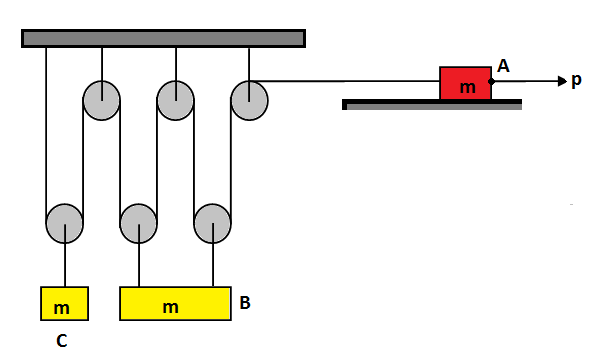# The block has unreliable power, it will not move...Three blocks $A,~B,C$ each of mass $m$ are arranged in pulley string system as shown in figure. All pulleys are massless and frictionless , while all strings are light and inextensible. Coefficient of kinetic friction between block $A$ and horizontal surface is equal to $0.5$ and force $P$ acts on it. The value of $\dfrac{p}{mg}$ for which block $C$ does not move, is equal to?

×